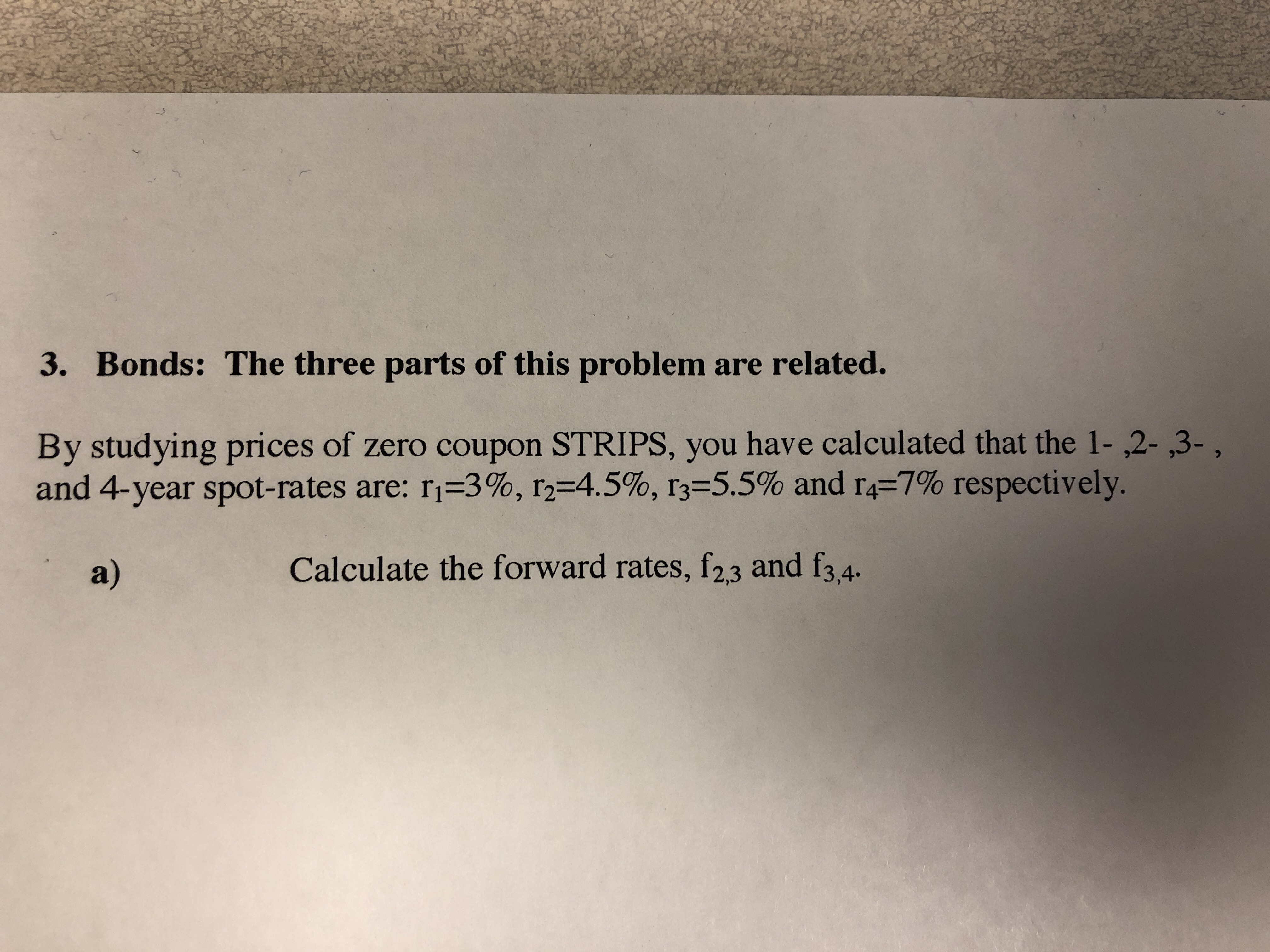# 3. Bonds: The three parts of this problem are related.By studying prices of zero coupon STRIPS, you have calculated that the 1- ,2- ,3-,and 4-year spot-rates are: ri-3%, 12-4.5 %, r3-5.5% and r4-7% respectively.a)Calculate the forward rates, f23 and f3,4.

Question
4 viewshelp_outlineImage Transcriptionclose3. Bonds: The three parts of this problem are related. By studying prices of zero coupon STRIPS, you have calculated that the 1- ,2- ,3-, and 4-year spot-rates are: ri-3%, 12-4.5 %, r3-5.5% and r4-7% respectively. a) Calculate the forward rates, f23 and f3,4. fullscreen
check_circle

star
star
star
star
star
1 Rating
Step 1

If rn andrSn+1 are the spot rates for the year n and n+1 respectively, then one year forward rate between year n and n+1 is given by fn, n+1 and governed by the following equation:

(1 + rn)n x (1 + fn, n+1) = (1 + rn + 1)n + 1

Step 2

In order to get f2, 3 we need to put n = 2. In that case, the equation becomes:

(1 + r2)2 x (1 + f2, 3) = (1 + r3)3

Or, (1 + 4.5%)2 x (1 + f2, 3) = (1 + 5.5%)3

Or, f2, 3 = (1 + 5.5%)3 / (1+ 4.5%)2 – 1 =  7.5288%

Step 3

In order to get f3, 4 we need to put n = 3. In that case, the equation becomes:

(1 + r3)3 x (1 + f3, 4) = (1 + r4)4

Or, (1 + 5.5%)3 x (1 + f3, 4) = (1 + 7%)4

Or, f3, ...

### Want to see the full answer?

See Solution

#### Want to see this answer and more?

Solutions are written by subject experts who are available 24/7. Questions are typically answered within 1 hour.*

See Solution
*Response times may vary by subject and question.
Tagged in